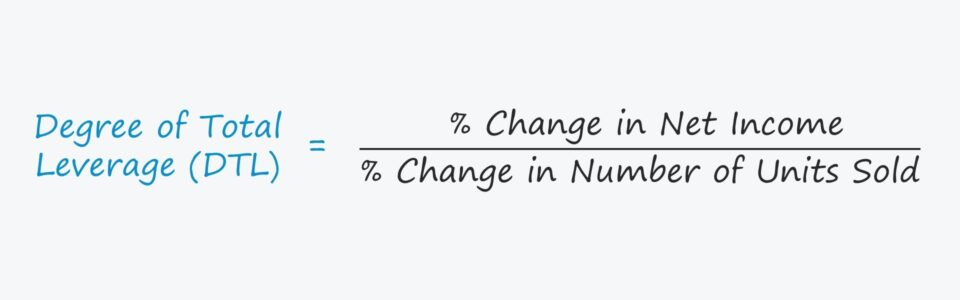# Degree of Total Leverage (DTL)

Understand the Degree of Total Leverage (DTL) Concept• What does the degree of total leverage (DTL) measure?
• What formula is used to calculate the degree of total leverage (DTL)?
• How is the degree of total leverage (DTL) related to financial leverage and operating leverage?
• What are the risks to a high degree of total leverage (DTL)?

## Degree of Total Leverage (DTL) Definition

The degree of total leverage (DTL) quantifies a company’s total leverage, which is composed of operating and financial leverage.

The degree of total leverage (DTL) refers to the sensitivity of a company’s net income, with respect to the number of units sold.

The DTL metric accounts for both the degree of operating leverage (DOL) and the degree of financial leverage (DFL).

1. Degree of Operating Leverage: DOL measures the proportion of a company’s cost structure that is comprised of fixed costs as opposed to variable costs.
2. Degree of Financial Leverage: DFL quantifies the sensitivity of net income (or EPS) is to changes in its operating profit (EBIT) that is attributable to debt financing (i.e. the fixed financing costs, namely interest expense).

The DTL can be interpreted as stating, “For each 1% change in number of units sold, the company’s net income will increase (or decrease) by ___%”.

The general guidelines for interpreting the two metrics are as follows:

• Degree of Operating Leverage (DOL): The greater the DOL, the more sensitive operating income (EBIT) is to changes in sales.
• Degree of Financing Leverage (DFL): The higher the DFL, the more sensitive that net income is to changes in operating income (EBIT).

The total leverage of a company — operating leverage and financial leverage — can contribute towards magnified earnings and profit margins, both positively and negatively.

## Degree of Total Leverage (DTL) Formula

One method to calculate the degree of total leverage (DTL) is to multiply the degree of operating leverage (DOL) by the degree of financial leverage (DFL).

###### DTL Formula
• Degree of Total Leverage (DTL) = Degree of Operating Leverage (DOL) × Degree of Financial Leverage (DFL)

Suppose a company has a degree of operating leverage (DOL) of 1.20x and a degree of financial leverage (DFL) of 1.25x.

The company’s degree of total leverage is equal to the product of DOL and DFL, which comes out to 1.50x

• Degree of Total Leverage (DTL) = 1.20x × 1.25x = 1.50x

## Degree of Total Leverage (DTL) Example Calculation

A different method to calculate the DTL consists of dividing the % change in net income by the % change in number of units sold.

###### DTL Formula
• Degree of Total Leverage (DTL) = % Change in Net Income ÷ % Change in Number of Units Sold

Suppose a company experienced an off-year, where sales declined by 4.0%.

If we assume the company’s DTL is 1.5x, the percentage change in net income can be calculated by re-arranging the formula from above.

DTL is equal to the % change in net income divided by the % change in units sold, so the implied % change in net income comes out to the % change in sales multiplied by the DTL.

• % Change in Net Income = –4.0% × 1.5x = –6.0%

## Degree of Total Leverage (DTL) Formula Breakdown

The final formula to calculate the degree of total leverage (DTL) that we’ll discuss is shown below.

###### DTL Formula
• DTL = Contribution Margin ÷ (Contribution Margin – Fixed Costs – Interest Expense)

The contribution margin equals “Quantity Sold × (Unit Price – Variable Cost Per Unit),” so the formula can be further expanded to:

###### DTL Formula
• DTL = Q (P – V) ÷ [Q (P – V) – FC – I]

Where:

• Q = Quantity Sold
• P = Unit Price
• V = Variable Cost Per Unit
• FC = Fixed Costs
• I = Interest Expense (Fixed Financial Costs)

For example, let’s assume that a company has sold 1,000 units at a unit price of \$5.00.

If the variable cost per unit is \$2.00, fixed costs are \$400, and interest expense is \$200, then the DTL is 1.25x.

• DTL = 1,000 (\$5.00 – \$2.00) ÷ [1,000 (\$5.00 – \$2.00) – \$400 – \$200)

Therefore, if the company were to sell 1% more units, its net income would be anticipated to rise by approximately 1.25%.Step-by-Step Online Course

#### Everything You Need To Master Financial Modeling

Enroll in The Premium Package: Learn Financial Statement Modeling, DCF, M&A, LBO and Comps. The same training program used at top investment banks.Inline FeedbacksLearn Financial Modeling Online

Everything you need to master financial and valuation modeling: 3-Statement Modeling, DCF, Comps, M&A and LBO.

X

The Wall Street Prep Quicklesson Series

7 Free Financial Modeling Lessons

Get instant access to video lessons taught by experienced investment bankers. Learn financial statement modeling, DCF, M&A, LBO, Comps and Excel shortcuts.